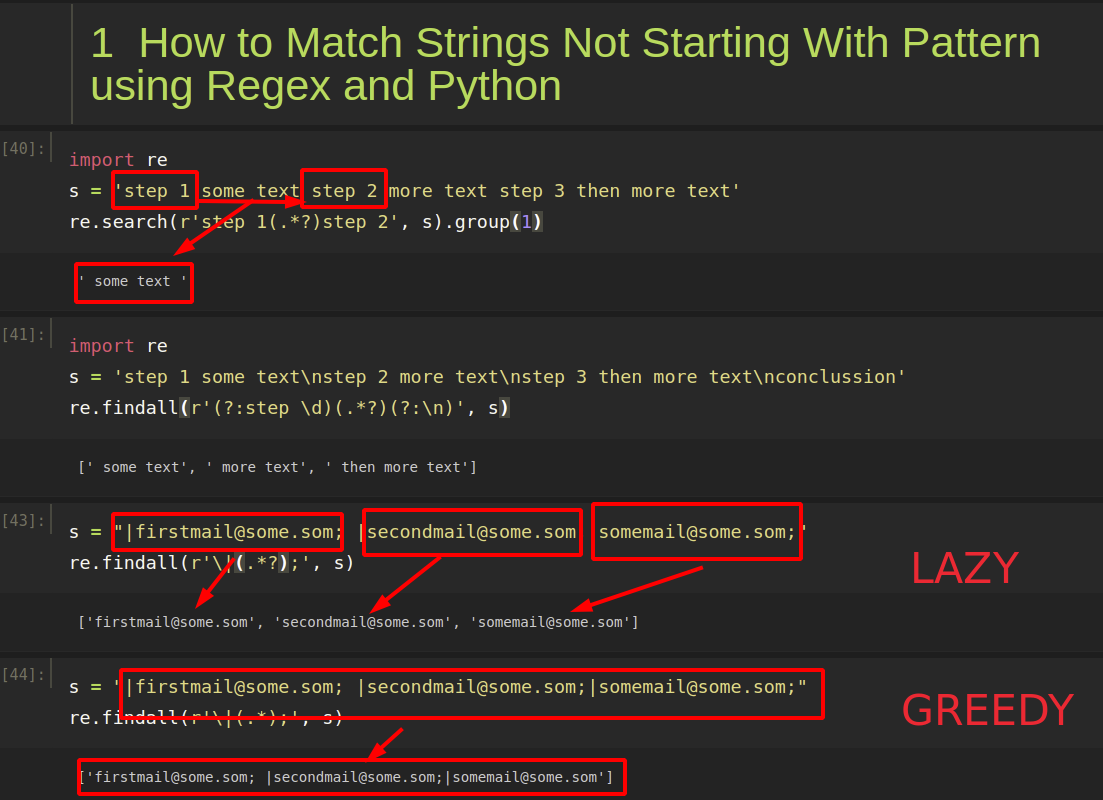To match any text between two strings/patterns with a regular expression in Python you can use:

``````re.search(r'pattern1(.*?)pattern2', s).group(1)
``````

In the next sections, you’ll see how to apply the above using a simple example.

## Step 1: Match Text Between Two Strings

To start with a simple example, let’s have a the next text:

step 1 some text step 2 more text step 3 then more text

and we would like to extract everything between `step 1` and `step 2`. To do so we are going to use capture group like:

``````import re
s = 'step 1 some text step 2 more text step 3 then more text'
re.search(r'step 1(.*?)step 2', s).group(1)
``````

result:

``````' some text '
``````

How it works:

• `step 1` - matches the characters step 1 literally (case sensitive)
• `(.*?)` - matches any character between zero and unlimited times expanding as needed (lazy)
• `step 2` - matches the characters step 2 literally (case sensitive)

### Non lazy search

The previous example will stop until it finds text which satisfies it. If you like to extract:

``````some text step 2 more text
``````

Then you need to change the search to:

``````re.findall(r'step \d (.*) step \d', s)
``````

## Step 2: Match Text Between Two Patterns

Now let's say that you would like to match a pattern and not fixed text. In this example we will see how to extract `step` followed by a digit:

``````import re
s = 'step 1 some text\nstep 2 more text\nstep 3 then more text\nconclusion'
re.findall(r'(?:step \d)(.*?)(?:\n)', s)
``````

So having the next text:

``````step 1 some text
step 2 more text
step 3 then more text
conclusion
``````

We will extract:

``````[' some text', ' more text', ' then more text']
``````

How does it work?

• `(?:step \d)` - Non-capturing group - `?:` - it will be matched but not extracted
• `step \d` - matches the characters step literally (case sensitive) followed by a digit (equivalent to [0-9])
• `(.*?)` - 1st Capturing Group - capture anything lazy mode
• `(?:\n)` - Non-capturing group
• `\n` matches a newline character

## Step 3: Match Text Between Two Patterns Lazy vs Greedy

In this step we will give a more explanation to the lazy vs greedy match. The difference can be explained as:

• `@(.*?)@` - Lazy
• `@(.*)@` - Greedy

So let say that we have a list of mails like:

If we do a greedy extraction between to strings we will get:

``````s = "|[email protected]; |[email protected];|[email protected];"
re.findall(r'\|(.*);', s)
``````

result will be only 1 match from the first `|` to the last `;`:

While if we do a lazy search for a text between two substrings then we will get:

``````s = 'step 1 some text\nstep 2 more text\nstep 3 then more text\nconclusion'
re.findall(r'(?:step \d)(.*?)(?:\n)', s)
``````

3 separate strings as:

[' some text', ' more text', ' then more text']